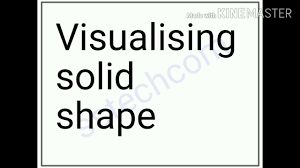# Visualizing Solid Shapes: Three-Dimensional Object or Shape Can Look Differently From Different Positions

In this quiz, you will find questions related to solid shapes like their edges, vertices, faces, etc.Start Quiz

What cross-section do you get when you give a horizontal cut to an ice-cream cone?

Triangle Circle Rectangle Square

What cross-section do you get when you give a horizontal cut to a brick?

Triangle Circle Square Rectangle

What cross-section do you get when you give a vertical cut to a brick?

Square Rectangle Triangle Circle

The number of edges of a cube is

8 12 6 3

If two cubes of dimensions 2 cm by 2 cm are placed side by side, what would the dimensions of resulting cuboid be ?

4, 2, 2 2, 4, 2 2, 2, 4 Noun of these

Which of the following is the number of vertices of sphere?

0 1 2 4

The number of triangular faces of a triangular prism is _______.

2 1 4 None of these

Three cubes each with 2 cm edge are placed side by side to form a cuboid. Its length will be :

4 cm 2 cm 6 cm None of these

The number of faces of a triangular pyramid or tetrahedron is _______.

4 6 5 1024

Two cubes of edge length 2 cm are placed side by side. The length of the resulting cuboid is

2 cm

4 cm

1 cm

None of these

Which one of the following is not a cuboid

Playing die

Geometry box

Briefcase

A box of corn flakes

Which one of the following is not a sphere

A ring

A cricket ball

A tennis ball

A football

The joint between separate faces of a solid is called an

Edge

Face

Vertex

Diagonal

Which of the following is a polyhedron

Prism

Cylinder

Cone

Cuboid

Which of the following has two edges and no corner

Cylinder

Prism

Cone

Sphere

Quiz/Test Summary
Title: Visualizing Solid Shapes: Three-Dimensional Object or Shape Can Look Differently From Different Positions
Questions: 15
Contributed by: